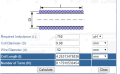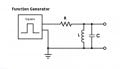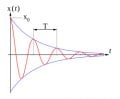# Measuring inductance with an oscilloscope.

#### dikidera

Joined Sep 6, 2015
14
I recently purchased a cheap chinese benchtop digital scope. I wanted to use it to measure inductance, by making an LC circuit and seeing the damped oscillations, measuring the distance of the period of the wave(aka the frequency).

I used the following formula: 1/sqr(2pi*f)*C, where C is a known capacitor. My setup consisted of me charging a capacitor, and then plugging in it in the LC configuration, I set my scope for single shot capture, I measured the resulting waveform, but according to the formula, it puts the inductor in the order of femtohenries. The inductor is a hand-made coil with several turns of a UTP cable, whose diameter is around 0.52mm. It should have been several hundred nanohenries, so I then used a known 100 microhenry inductor(the type with 4 color code bands), and a 1000 microfarad(electrolytic) capacitor, but the resulting frequency, was around 1.5mhz, rather than the 0.0005033mhz that it should have been. I also tried using a 1 microfarad film capacitor, but the frequency was still wrong..

Perhaps I've hooked it up wrong? I place the oscilloscope probe on one end, and the ground probe to the other end of the circuit.

The scope is not the best, it is a Hantek DSO5102P, 100Mhz BW(says 1Gsa/s) but with a rather small memory of 40 kilopoints.

Last edited:

#### dikidera

Joined Sep 6, 2015
14
Huh? I know of the sound card oscilloscope, but why would I use it, when I have a dedicated benchtop scope?

#### MrChips

Joined Oct 2, 2009
20,185
All you need is a series resistor and a square-wave signal. You can use a 555-timer circuit to generate the square wave or you can use the 1kHz test signal from the PROBE COMP output from the oscilloscope.And measure the time constant t = L / R.#### dikidera

Joined Sep 6, 2015
14
But the inductor is an unknown value. It is what I wish to find out.

#### MrChips

Joined Oct 2, 2009
20,185
If you measure time-constant and you know R, the formula for inductance L is

L = R x time-constant

Joined Mar 10, 2018
3,883
Huh? I know of the sound card oscilloscope, but why would I use it, when I have a dedicated benchtop scope?
My post was targeted at link for sound card impedance bridge, I should

Regards, Dana.

#### dikidera

Joined Sep 6, 2015
14
If I measure between the R and L node, I get no wave at all. If I place the probe before the resistor, thats when I get a waveform. Is this correct?

#### MrChips

Joined Oct 2, 2009
20,185
If I measure between the R and L node, I get no wave at all. If I place the probe before the resistor, thats when I get a waveform. Is this correct?
It would depend on the values of R, L, and the frequency of the square wave.

What is the expected value of L?

#### dikidera

Joined Sep 6, 2015
14
So I did some measurements. Between 0 and rise, the time was around 9 microseconds, 63% off that is 5.67. My resistor's value was 691K, so 691000*0,00000567(5.67 microseconds)=3,91797. How should I interpret this number? The expected value of the coil should be 0.00000121(lets add 5-10% error on that one) nanohenry.

#### MrChips

Joined Oct 2, 2009
20,185
Time-constant is the time it takes the voltage to reach 63% of the maximum voltage, not 63% of the period.One other complication is we need to know how the internal resistance of the signal source is going to affect the measurement.

#### MrChips

Joined Oct 2, 2009
20,185
I have determined that the method I suggested will not work for a number of reasons:

1) The resistor R has to be at least 10 times the internal resistance of the signal source.

2) The rise and fall times of the signal must be much shorter than the time-constants you are trying to determine.

3) An inductor of 100nH makes it difficult to get meaningful results because of 1) and 2) above.

#### BR-549

Joined Sep 22, 2013
4,938

#### dikidera

Joined Sep 6, 2015
14
Is it not possible to measure the inductance with a simple LC circuit, without a function generator. Simply charging the cap, and inserting it into the circuit, while the scope is on single trigger mode.

I tried this method(my triggering is either bad or the methodology is wrong), but I was able to, after 30-40 cap charges, to observe only TWICE, a damped oscillation.

However that damped oscillation put my coil at around 600 nanohenry, while it was designed to be 1200, which is a 50% difference.

My coil is an 8 turn UTP cable, 9.93 diameter of the coil, and the diameter of the wire is ~0.52mm, which puts it at around 1210 nanohenry or 1.2 microHenry. On my 1uF film cap, with this coil I observed an oscillation of around 200khz, which puts the coil at 600 nanohenry rather than 1210.

Joined Dec 4, 2009
328
For the parameters given, 9.93mm dia, and dia of the wire ~0.52mm, if the coil is would without any gap gives the length of the coil of ~4.16mm. And that gives an inductance of about 750nH. Providing for some gap between the turns, the length of the coil would increase, and 600nH appears correct to me. Please check the calculations.

#### dikidera

Joined Sep 6, 2015
14

Joined Dec 4, 2009
328Thanks, it never occurred to me that there are calculators here itself!

I used the following ones.
http://www.circuits.dk/calculator_single_layer_aircore.htm
http://www.daycounter.com/Calculators/Air-Core-Inductor-Calculator.phtml

Both gave similar results. I have used the daycounter calculator earlier and found it to be correct.
If the coil length is increased, that is stretch the coil, then the inductance goes down. I do not see this parameter in the equation in AAC.
If you use the 0.52mm (dia of the wire) as the length of the coil in the links above, then the calculations match with AAC. But then the length of the coil is at least 8 times the wire dia, not taking the gaps between the windings into account.

#### MrChips

Joined Oct 2, 2009
20,185
You can try using any low frequency square-wave pulse into L and C parallel resonance circuit.Measure the period of the damped oscillation.With C = 100nF and L = 1000nH,
resonance frequency = 500kHz, period T = 2μs
Very doable on an oscilloscope.

C = 100nF and L = 600nH
f = 650kHz
T = 1.54μs

#### MrAl

Joined Jun 17, 2014
7,106
I recently purchased a cheap chinese benchtop digital scope. I wanted to use it to measure inductance, by making an LC circuit and seeing the damped oscillations, measuring the distance of the period of the wave(aka the frequency).

I used the following formula: 1/sqr(2pi*f)*C, where C is a known capacitor. My setup consisted of me charging a capacitor, and then plugging in it in the LC configuration, I set my scope for single shot capture, I measured the resulting waveform, but according to the formula, it puts the inductor in the order of femtohenries. The inductor is a hand-made coil with several turns of a UTP cable, whose diameter is around 0.52mm. It should have been several hundred nanohenries, so I then used a known 100 microhenry inductor(the type with 4 color code bands), and a 1000 microfarad(electrolytic) capacitor, but the resulting frequency, was around 1.5mhz, rather than the 0.0005033mhz that it should have been. I also tried using a 1 microfarad film capacitor, but the frequency was still wrong..

Perhaps I've hooked it up wrong? I place the oscilloscope probe on one end, and the ground probe to the other end of the circuit.

The scope is not the best, it is a Hantek DSO5102P, 100Mhz BW(says 1Gsa/s) but with a rather small memory of 40 kilopoints.
Hello there,

The measurement depends on what it is going to be used for and what type of inductor it is.

The best measurement is obtained by placing the inductor into the circuit in which it will be used for especially of the core is not air. That's because the inductance changes with DC bias, and a lot of circuits show significant DC bias in the applications.
DC-DC converters are especially of concern here because there is always a DC bias. The DC bias causes the inductance to fall. The inductance can change more than 50 percent in some cases as the DC current changes. The so called "swinging chokes" are also designed to change inductance as the current goes up in order to increase efficiency.

The BH curve tells us that the permeability starts out somewhat low, then climbs up to some nominal value which we usually associate with the normal inductance value, then it starts to decrease again and stays lower as the DC bias increases. Eventually the construction starts to look something like an air core coil because the core is no longer effective in boosting the inductance of the coil of wire around it.

You can still get an idea what you have there by using the same test for every inductor you use, but you should still gear it for the application. For example, for an inductor that will be used in a DC converter you can use a square wave and use the formula:
v=L*di/dt
and this requires measuring the current and time and using a known square wave voltage source that is capable of driving the inductor properly.
If you look at this test and say a buck converter, you'll see similar results, except in the buck converter we have a means right there to measure the inductor with various DC bias just by changing the load resistance value. That changes the DC bias of the coil and thus allows us to get several measurements with low current and with maximum current and anything in between.
If you intend to use it in an oscillator circuit, you should try to measure the inductance while it is running.